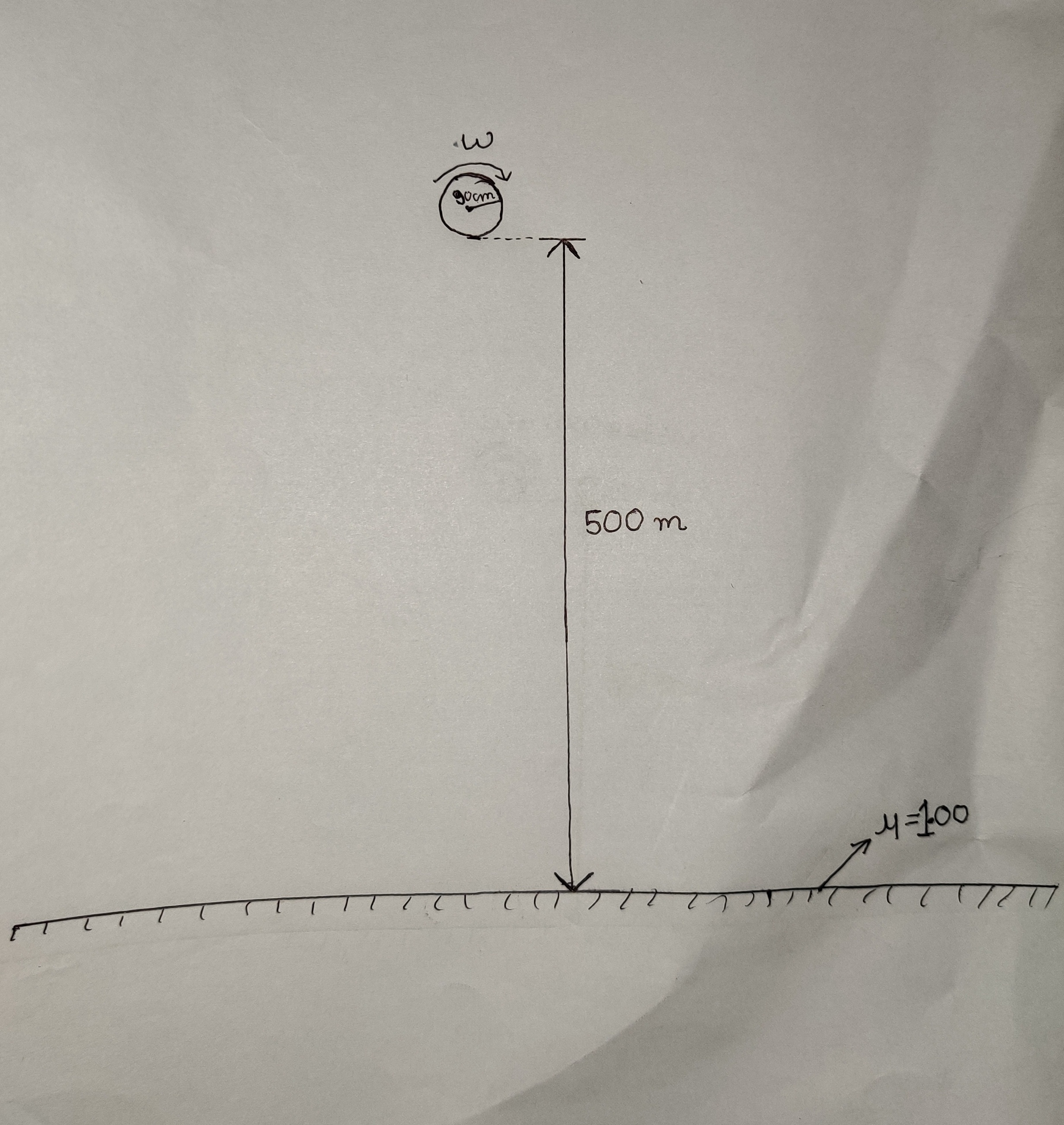# Bouncy Bouncing Ball!From a height $h = 500 \text{ m}$, a uniform solid disc of radius $R = 90 \text{ cm}$ is given an angular velocity $\omega = \dfrac{5000}{3} \text{ rad/s}$ and dropped. The ground is rough and has coefficient of friction $\mu = 1$. After each collision, the disc's vertical speed becomes half$(50\%)$ of the value just before collision. If when the vertical velocity just becomes zero, the horizontal distance travelled by disc is $r (\text{in m})$ and the angular velocity of disc is $\omega' (\text{in rad}/\text{s})$, enter answer as $r + \omega'$.

Take acceleration due to gravity $g = 10\text{ m/s}^2$.

Inspiration Aniket Sanghi

All of my problems are original.

×

Problem Loading...

Note Loading...

Set Loading...